您可以捐助，支持我们的公益事业。 1元 10元 50元 认证码：必填求知 文章 文库 Lib 视频 iPerson 课程 认证 咨询 工具 讲座 Modeler Code要资料订阅捐助
GAFT：一个使用Python实现的遗传算法框架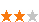2083 次浏览     评价： 好 中 差

GitHub: https://github.com/PytLab/gaft

PyPI: https://pypi.python.org/pypi/gaft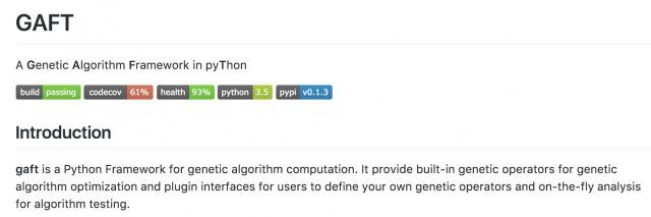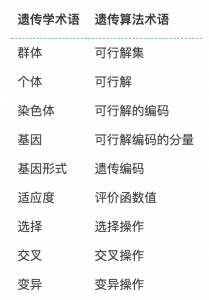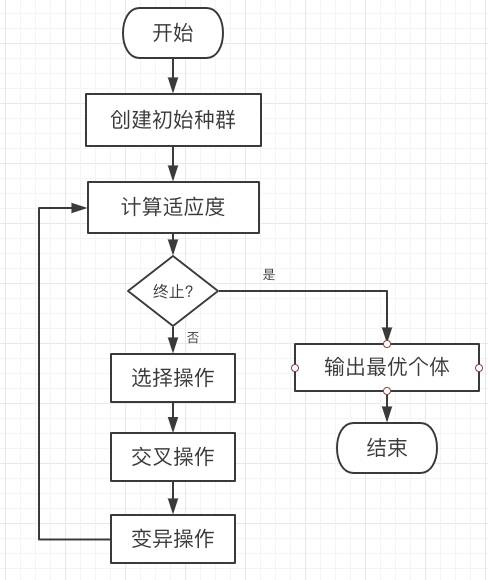gaft 设计原则

GAFT文件结构

 . ├── LICENSE ├── MANIFEST.in ├── README.rst ├── examples │ ├── ex01 │ └── ex02 ├── gaft │ ├── __init__.py │ ├── __pycache__ │ ├── analysis │ ├── components │ ├── engine.py │ ├── operators │ └── plugin_interfaces ├── setup.cfg ├── setup.py └── tests ├── flip_bit_mutation_test.py ├── gaft_test.py ├── individual_test.py ├── population_test.py ├── roulette_wheel_selection_test.py └── uniform_crossover_test.py

/gaft/components中定义了内置的个体和种群类型，提供了两种不同的遗传编码方式:二进制编码和实数编码。

/gaft/plugin_interfaces中是插件接口定义，所有的算子定义以及on-the-fly分析的接口规则都在里面，使用者可以根据此来编写自己的插件并放入到engine中。

/gaft/operators里面是内置遗传算子，他们也是遵循/gaft/plugin_interfaces中的规则进行编写，可以作为编写算子的例子。其中算子我目前内置了roulette wheel选择算子，uniform 交叉算子和flipbit变异算子，使用者可以直接使用内置算子来使用gaft对自己的问题进行优化。

/gaft/analysis里面是内置的on-the-fly分析插件，他可以在遗传算法迭代的过程中对迭代过程中的变量进行分析，例如我在里面内置了控制台日志信息输出，以及迭代适应度值的保存等插件方便对进化曲线作图。

/gaft/engine便是遗传算法的流程控制模块了，他将所有的之前定义的各个部分组合到一起使用遗传算法流程进行优化迭代。

1. 先导入需要的模块

 from math import sin, cos # 导入种群和内置算子相关类 from gaft import GAEngine from gaft.components import GAIndividual from gaft.components import GAPopulation from gaft.operators import RouletteWheelSelection from gaft.operators import UniformCrossover from gaft.operators import FlipBitMutation # 用于编写分析插件的接口类 from gaft.plugin_interfaces.analysis import OnTheFlyAnalysis # 内置的存档适应度函数的分析类 from gaft.analysis.fitness_store import FitnessStoreAnalysis # 我们将用两种方式将分析插件注册到遗传算法引擎中

2. 创建引擎

 # 定义种群 indv_template = GAIndividual(ranges=[(0, 10)], encoding='binary', eps=0.001) population = GAPopulation(indv_template=indv_template, size=50) # 创建遗传算子 selection = RouletteWheelSelection() crossover = UniformCrossover(pc=0.8, pe=0.5) mutation = FlipBitMutation(pm=0.1) # 创建遗传算法引擎, 分析插件和适应度函数可以以参数的形式传入引擎中 engine = GAEngine(population=population, selection=selection, crossover=crossover, mutation=mutation, analysis=[FitnessStoreAnalysis])

3. 自定义适应度函数

 @engine.fitness_register def fitness(indv): x, = indv.variants return x + 10*sin(5*x) + 7*cos(4*x)

4. 自定义on-the-fly分析插件

 @engine.analysis_register class ConsoleOutputAnalysis(OnTheFlyAnalysis): interval = 1 def register_step(self, ng, population, engine): best_indv = population.best_indv(engine.fitness) msg = 'Generation: {}, best fitness: {:.3f}'.format(ng, engine.fitness(best_indv)) engine.logger.info(msg) def finalize(self, population, engine): best_indv = population.best_indv(engine.fitness) x = best_indv.variants y = engine.fitness(best_indv) msg = 'Optimal solution: ({}, {})'.format(x, y) engine.logger.info(msg)

5. Ok, 开始跑(优化)吧!

 if '__main__' == __name__: # Run the GA engine. engine.run(ng=100)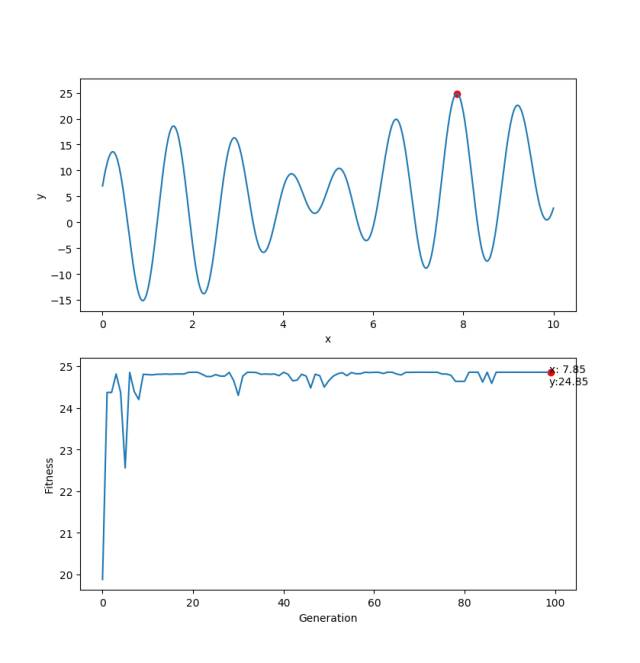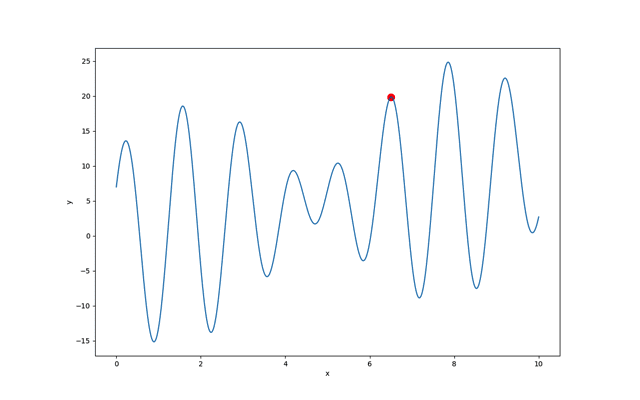''' Find the global maximum for binary function: f(x) = y*sim(2*pi*x) + x*cos(2*pi*y) ''' from math import sin, cos, pi from gaft import GAEngine from gaft.components import GAIndividual from gaft.components import GAPopulation from gaft.operators import RouletteWheelSelection from gaft.operators import UniformCrossover from gaft.operators import FlipBitMutation # Built-in best fitness analysis. from gaft.analysis.fitness_store import FitnessStoreAnalysis from gaft.analysis.console_output import ConsoleOutputAnalysis # Define population. indv_template = GAIndividual(ranges=[(-2, 2), (-2, 2)], encoding='binary', eps=0.001) population = GAPopulation(indv_template=indv_template, size=50) # Create genetic operators. selection = RouletteWheelSelection() crossover = UniformCrossover(pc=0.8, pe=0.5) mutation = FlipBitMutation(pm=0.1) # Create genetic algorithm engine. # Here we pass all built-in analysis to engine constructor. engine = GAEngine(population=population, selection=selection, crossover=crossover, mutation=mutation, analysis=[ConsoleOutputAnalysis, FitnessStoreAnalysis]) # Define fitness function. @engine.fitness_register def fitness(indv): x, y = indv.variants return y*sin(2*pi*x) + x*cos(2*pi*y) if '__main__' == __name__: engine.run(ng=100)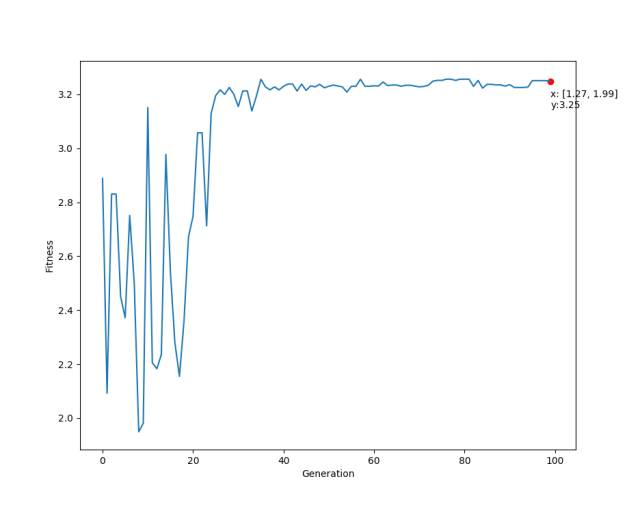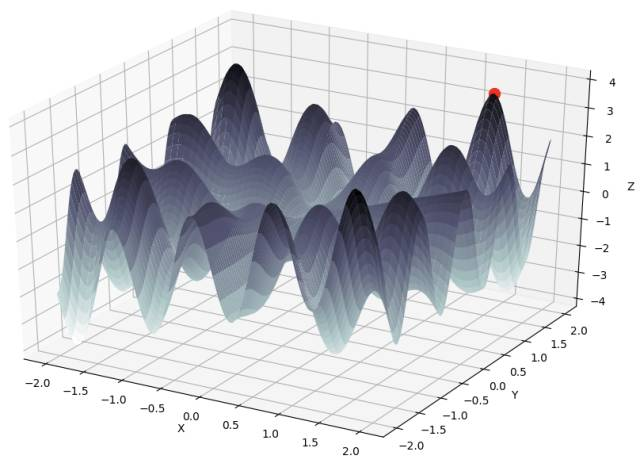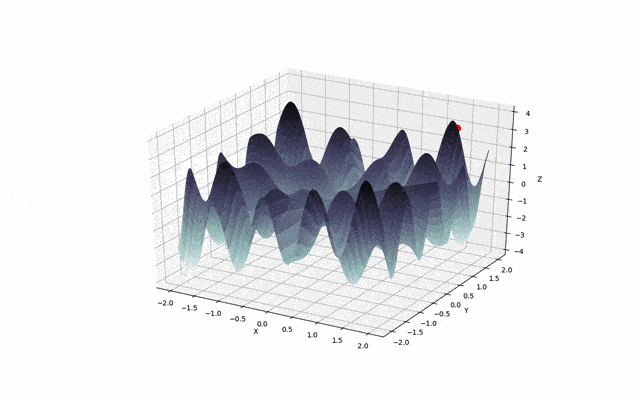2083 次浏览     评价： 好 中 差订阅捐助
 相关文章 手机软件测试用例设计实践 手机客户端UI测试分析 iPhone消息推送机制实现与探讨 Android手机开发（一）
 相关文档 Android_UI官方设计教程 手机开发平台介绍 android拍照及上传功能 Android讲义智能手机开发
 相关课程 Android高级移动应用程序 Android系统开发 Android应用开发 手机软件测试